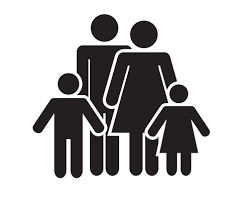# Large family

I have as many brothers as sisters, and each of my brothers has twice as many sisters as brothers. How many children do parents have?

n =  7

### Step-by-step explanation:

n = m+z
m -1 = z
2(z - 1) = m

n = m+z
m -1 = z
2·(z - 1) = m

m-n+z = 0
m-z = 1
m-2z = -2

Row 2 - Row 1 → Row 2
m-n+z = 0
n-2z = 1
m-2z = -2

Row 3 - Row 1 → Row 3
m-n+z = 0
n-2z = 1
n-3z = -2

Row 3 - Row 2 → Row 3
m-n+z = 0
n-2z = 1
-z = -3

z = -3/-1 = 3
n = 1+2z = 1+2 · 3 = 7
m = 0+n-z = 0+7-3 = 4

m = 4
n = 7
z = 3

Our linear equations calculator calculates it.Did you find an error or inaccuracy? Feel free to write us. Thank you!

Tips for related online calculators
Do you have a linear equation or system of equations and looking for its solution? Or do you have a quadratic equation?